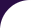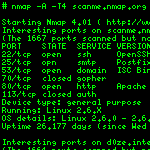Intro Reference Guide Book Install Guide Download Changelog Zenmap GUI Docs Bug Reports OS Detection Propaganda Related Projects In the Movies In the News# Library `listop`

Functional-style list operations.

People used to programming in functional languages, such as Lisp or Haskell, appreciate their handling of lists very much. The `listop` module tries to bring much of the functionality from functional languages to Lua using Lua's central data structure, the table, as a base for its list operations. Highlights include a `map` function applying a given function to each element of a list.

## Functions

 append (l1, l2) Concatenate two lists and return the result. apply (f, l) Calls the function with all the elements in the list as the parameters. car (l) Fetch the first element of a list. cdr (l) Fetch all elements following the first in a new list. cons (v1, v2) Prepend a value or list to another value or list. filter (f, l) Returns a list containing only those elements for which a predicate function returns true. flatten (l) Return a flattened version of a list. The flattened list contains only non-list values. is_empty (l) Returns true if the given list is empty. is_list (l) Returns true if the given value is a list (or rather a table). map (f, l) Calls `f` for each element in the list. The returned list contains the results of each function call. ncar (l, x) Fetch element at index `x` from `l`. ncdr (l, x) Fetch all elements following the element at index `x`. reverse (l) Return a list in reverse order.

## Functions

append (l1, l2)

Concatenate two lists and return the result.

• l1: List.
• l2: List.

### Return value:

List.
apply (f, l)

Calls the function with all the elements in the list as the parameters.

### Parameters

• f: The function to call.
• l: A list.

### Usage:

`listop.apply(math.max,{1,5,6,7,50000}) --> 50000`

### Return value:

Results from `f`.
car (l)

Fetch the first element of a list.

• l: The list.

### Return value:

The first element.
cdr (l)

Fetch all elements following the first in a new list.

• l: The list.

### Return value:

Elements after the first.
cons (v1, v2)

Prepend a value or list to another value or list.

### Parameters

• v1: value or list.
• v2: value or list.

### Return value:

New list.
filter (f, l)

Returns a list containing only those elements for which a predicate function returns true.

The predicate has to be a function taking one argument and returning a Boolean. If it returns true, the argument is appended to the return value of filter.

### Parameters

• f: The function.
• l: The list.

### Usage:

`listop.filter(isnumber,{1,2,3,"foo",4,"bar"}) --> {1,2,3,4}`

### Return value:

Filtered list.
flatten (l)

Return a flattened version of a list. The flattened list contains only non-list values.

### Parameters

• l: The list to flatten.

### Usage:

`listop.flatten({1,2,3,"foo",{4,5,{"bar"}}}) --> {1,2,3,"foo",4,5,"bar"}`

### Return value:

Flattened list.
is_empty (l)

Returns true if the given list is empty.

• l: A list.

### Return value:

True or false.
is_list (l)

Returns true if the given value is a list (or rather a table).

### Parameters

• l: Any value.

### Return value:

True or false.
map (f, l)

Calls `f` for each element in the list. The returned list contains the results of each function call.

### Parameters

• f: The function to call.
• l: A list.

### Usage:

`listop.map(tostring,{1,2,true}) --> {"1","2","true"}`

### Return value:

List of function results.
ncar (l, x)

Fetch element at index `x` from `l`.

### Parameters

• l: The list.
• x: Element index.

### Return value:

Element at index `x` or at index `1` if `x` is not given.
ncdr (l, x)

Fetch all elements following the element at index `x`.

### Parameters

• l: The list.
• x: Element index.

### Return value:

Elements after index `x` or after index `1` if `x` is not given.
reverse (l)

Return a list in reverse order.

• l: List.

Reversed list.